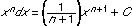# integration

﻿
integration
/in'ti gray"sheuhn/, n.
1. an act or instance of combining into an integral whole.
2. an act or instance of integrating a racial, religious, or ethnic group.
3. an act or instance of integrating an organization, place of business, school, etc.
4. Math. the operation of finding the integral of a function or equation, esp. solving a differential equation.
5. behavior, as of an individual, that is in harmony with the environment.
6. Psychol. the organization of the constituent elements of the personality into a coordinated, harmonious whole.
7. Genetics. coadaptation (def. 2).
[1610-20; INTEGRATE + -ION; cf. L integratio renewal]
Syn. 1. combination, blending, fusing.

* * *

In calculus, the process of finding a function whose derivative is a given function.

The term, sometimes used interchangeably with "antidifferentiation," is indicated symbolically with the integral sign ∫. (The differential dx usually follows to indicate x as the variable.) The basic rules of integration are: (1) ∫(f + g)dx = ∫fdx + ∫gdx (where f and g are functions of the variable x), (2) ∫kfdx = kfdx (k is a constant), and (3)(C is a constant). Note that any constant value may be added onto an indefinite integral without changing its derivative. Thus, the indefinite integral of 2x is x2 + C, where C can be any real number. A definite integral is an indefinite integral evaluated over an interval. The result is not affected by the choice for the value of C. See also differentiation.

* * *

in mathematics, technique of finding a function g(x) the derivative of which, Dg(x), is equal to a given function f(x). This is indicated by the integral sign “∫,” as in ∫f(x), usually called the indefinite integral of the function. (The symbol dx is usually added, which merely identifies x as the variable.) The definite integral, written

with a and b called the limits of integration, is equal to g(b) − g(a), where Dg(x) = f(x).

Some antiderivatives can be calculated by merely recalling which function has a given derivative, but the techniques of integration mostly involve classifying the functions according to which types of manipulations will change the function into a form the antiderivative of which can be more easily recognized. For example, if one is familiar with derivatives, the function 1/(x + 1) can be easily recognized as the derivative of loge(x + 1). The antiderivative of (x2 + x + 1)/(x + 1) cannot be so easily recognized, but if written as x(x + 1)/(x + 1) + 1/(x + 1) = x + 1/(x + 1), it then can be recognized as the derivative of x2/2 + loge(x + 1). One useful aid for integration is the theorem known as integration by parts. In symbols, the rule is ∫fDg = fg − ∫gDf. That is, if a function is the product of two other functions, f and one that can be recognized as the derivative of some function g, then the original problem can be solved if one can integrate the product gDf. For example, if f = x, and Dg = cos x, then ∫x·cos x = x·sin x − ∫sin x = x·sin x − cos x + C. Integrals are used to evaluate such quantities as area, volume, work, and, in general, any quantity that can be interpreted as the area under a curve.

* * *

Universalium. 2010.

### Look at other dictionaries:

• Integration — Integration …   Deutsch Wörterbuch

• intégration — [ ɛ̃tegrasjɔ̃ ] n. f. • 1700; « rétablissement » 1309; lat. integratio → intégrer 1 ♦ Math. Opération (inverse de la différentiation) par laquelle on détermine la grandeur limite de la somme de quantités infinitésimales en nombre indéfiniment… …   Encyclopédie Universelle

• integration — in‧te‧gra‧tion [ˌɪntˈgreɪʆn] noun [uncountable] 1. the combining of two or more organizations, activities etc so that they become more effective, make better profits etc: • The important achievement of the European Union is economic integration …   Financial and business terms

• Integration — is a process of combining or accumulating. It may specifically refer to:*The bringing together of two or more economies, cultures, religions (usually called syncretism), etc.;in sociology and economy *Social integration *Racial integration,… …   Wikipedia

• Integration — Intégration Cette page d’homonymie répertorie les différents sujets et articles partageant un même nom …   Wikipédia en Français

• integration — in·te·gra·tion n: the act or process or an instance of integrating: as a: a writing that embodies a complete and final agreement between parties b: incorporation as equals into society or an organization of individuals of different groups (as… …   Law dictionary

• Integration — In te*gra tion ([i^]n t[ e]*gr[=a] sh[u^]n), n. [L. integratio a renewing, restoring: cf. F. int[ e]gration.] [1913 Webster] 1. The act or process of making whole or entire. [1913 Webster] 2. (Math.) The operation of finding the primitive… …   The Collaborative International Dictionary of English

• integration — (n.) 1610s, from Fr. intégration and directly from L. integrationem (nom. integratio) renewal, restoration, noun of action from pp. stem of integrare (see INTEGRATE (Cf. integrate)). Anti discrimination sense is recorded from 1940 in a S.African… …   Etymology dictionary

• integration — integration. См. интеграция. (Источник: «Англо русский толковый словарь генетических терминов». Арефьев В.А., Лисовенко Л.А., Москва: Изд во ВНИРО, 1995 г.) …   Молекулярная биология и генетика. Толковый словарь.

• integration — articulation, concatenation (see under INTEGRATE vb) Analogous words: unification, consolidation, concentration (see corresponding verbs at COMPACT): integrity, union, *unity, solidarity …   New Dictionary of Synonyms

• integration — ► NOUN 1) the action or process of integrating. 2) the intermixing of peoples or groups previously segregated. DERIVATIVES integrationist noun …   English terms dictionary